Main Content

# tiledlayout

Create tiled chart layout

## Syntax

``tiledlayout(m,n)``
``tiledlayout('flow')``
``tiledlayout(___,Name,Value)``
``tiledlayout(parent,___)``
``t = tiledlayout(___)``

## Description

example

````tiledlayout(m,n)` creates a tiled chart layout for displaying multiple plots in the current figure. The layout has a fixed `m`-by-`n` tile arrangement that can display up to `m*n` plots. If there is no figure, MATLAB® creates a figure and places the layout into it. If the current figure contains an existing axes or layout, MATLAB replaces it with a new layout.A tiled chart layout contains an invisible grid of tiles that covers the entire figure or parent container. Each tile can contain an axes for displaying a plot. After creating a layout, call the `nexttile` function to place an axes object into the layout. Then call a plotting function to plot into the axes.```

example

````tiledlayout('flow')` specifies the `'flow'` tile arrangement for the layout. Initially, there is only one empty tile that fills the entire layout. As you call `nexttile`, the layout reflows as needed to accommodate the new axes while maintaining an aspect ratio of roughly 4:3 for all the tiles.```

example

````tiledlayout(___,Name,Value)` specifies additional options for the layout using one or more name-value pair arguments. Specify the options after all other input arguments. For example, `tiledlayout(2,2,'TileSpacing','compact')` creates a 2-by-2 layout with minimal spacing between the tiles. For a list of properties, see TiledChartLayout Properties.```

example

````tiledlayout(parent,___)` creates the layout in the specified parent container rather than in the current figure. Specify the parent container before all other input arguments.```

example

````t = tiledlayout(___)` returns the `TiledChartLayout` object. Use `t` to configure properties of the layout after creating it.```

## Examples

collapse all

Create a `2`-by-`2` tiled chart layout, and call the `peaks` function to get the coordinates of a predefined surface. Create an axes object in the first tile by calling the `nexttile` function. Then call the `surf `function to plot into the axes. Repeat the process using different plotting functions for the other three tiles.

```tiledlayout(2,2); [X,Y,Z] = peaks(20); % Tile 1 nexttile surf(X,Y,Z) % Tile 2 nexttile contour(X,Y,Z) % Tile 3 nexttile imagesc(Z) % Tile 4 nexttile plot3(X,Y,Z)```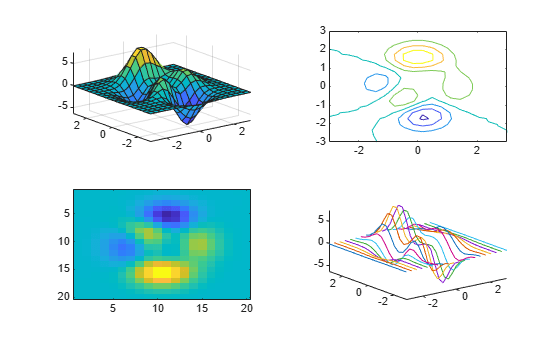Create four coordinate vectors: `x`, `y1`, `y2`, and `y3`. Call the `tiledlayout` function with the `'flow'` argument to create a tiled chart layout that can accommodate any number of axes. Call the `nexttile` function to create the first axes. Then plot `y1` in the first tile. This first plot fills the entire layout.

```x = linspace(0,30); y1 = sin(x/2); y2 = sin(x/3); y3 = sin(x/4); % Plot into first tile three times tiledlayout('flow') nexttile plot(x,y1)```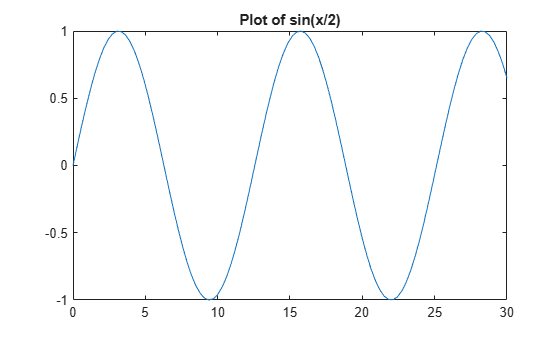Create a second tile and axes, and plot into the axes.

```nexttile plot(x,y2)```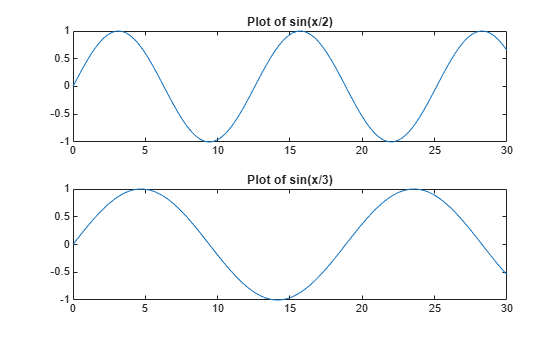Repeat the process to create a third plot.

```nexttile plot(x,y3)```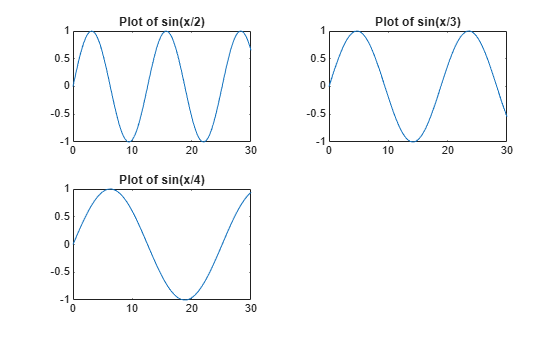Repeat the process to create a fourth plot. This time, plot all three lines in the same axes by calling `hold on` after plotting `y1`.

```nexttile plot(x,y1) hold on plot(x,y2) plot(x,y3) hold off```Create five coordinate vectors: `x`, `y1`, `y2`, `y3`, and `y4`. Then call the `tiledlayout` function to create a `2`-by-`2` layout and specify a return argument to store the `TileChartLayout` object. Call the `nexttile` function to create an axes object in the next empty tile before calling the `plot` function.

```x = linspace(0,30); y1 = sin(x); y2 = sin(x/2); y3 = sin(x/3); y4 = sin(x/4); t = tiledlayout(2,2); % Tile 1 nexttile plot(x,y1) % Tile 2 nexttile plot(x,y2) % Tile 3 nexttile plot(x,y3) % Tile 4 nexttile plot(x,y4)```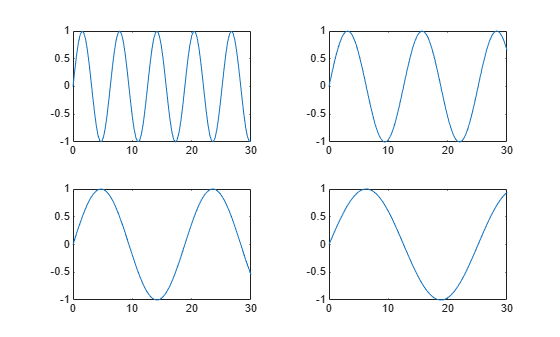Decrease the amount of space between the tiles by setting the `TileSpacing` property to `'compact'`. Then decrease the space between the edges of the layout and the edges of the figure by setting the `Padding` property to `'compact'`.

```t.TileSpacing = 'compact'; t.Padding = 'compact';```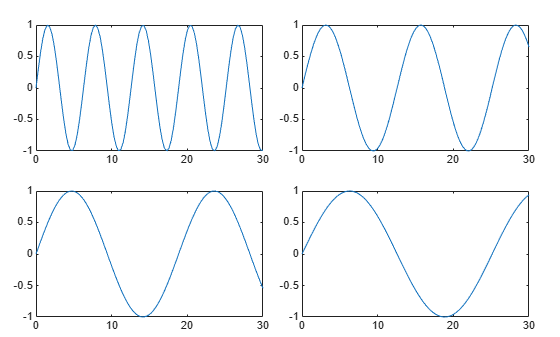Create a `2`-by-`2` tiled chart layout `t`. Specify the `TileSpacing` name-value pair argument to minimize the space between the tiles. Then create a titled plot in each tile.

```t = tiledlayout(2,2,'TileSpacing','Compact'); % Tile 1 nexttile plot(rand(1,20)) title('Sample 1') % Tile 2 nexttile plot(rand(1,20)) title('Sample 2') % Tile 3 nexttile plot(rand(1,20)) title('Sample 3') % Tile 4 nexttile plot(rand(1,20)) title('Sample 4')```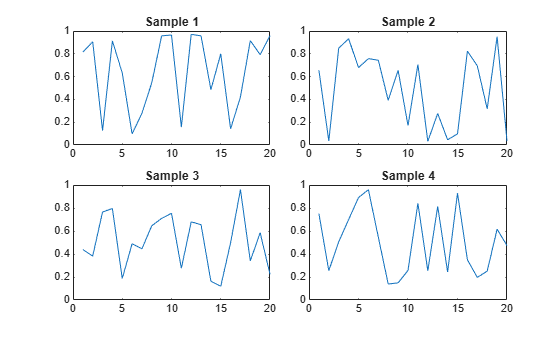Display a shared title and axis labels by passing `t` to the `title`, `xlabel`, and `ylabel` functions.

```title(t,'Size vs. Distance') xlabel(t,'Distance (mm)') ylabel(t,'Size (mm)')```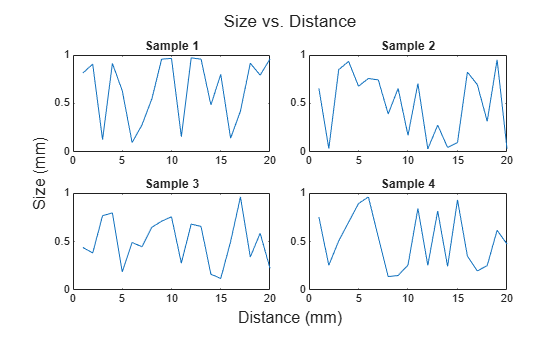Create a panel in a figure. Then create a tiled chart layout in the panel by specifying the panel object as the first argument to the `tiledlayout` function. Display a plot in each tile.

```p = uipanel('Position',[.1 .2 .8 .6]); t = tiledlayout(p,2,1); % Tile 1 nexttile(t) stem(1:13) % Tile 2 nexttile(t) bar([10 22 31 43 52])```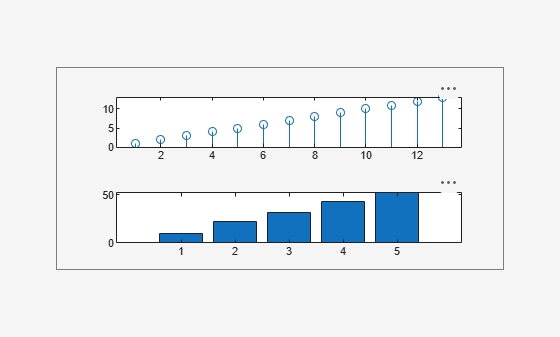Call the `tiledlayout` function to create a `2`-by-`1` tiled chart layout. Call the `nexttile` function with an output argument to store the axes. Then plot into the axes, and set the x- and y-axis colors to red. Repeat the process in the second tile.

```t = tiledlayout(2,1); % First tile ax1 = nexttile; plot([1 2 3 4 5],[11 6 10 4 18]); ax1.XColor = [1 0 0]; ax1.YColor = [1 0 0]; % Second tile ax2 = nexttile; plot([1 2 3 4 5],[5 1 12 9 2],'o'); ax2.XColor = [1 0 0]; ax2.YColor = [1 0 0];```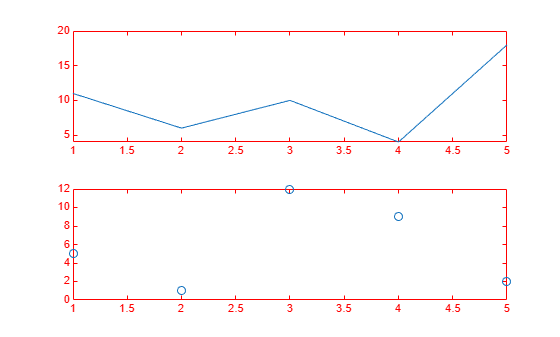To span an axes object from a specific location, specify the tile number and the span value.

Define `scores` and `strikes` as vectors containing bowling league data over four games. Then create a `3`-by-`3` tiled chart layout and display five bar graphs showing the number of strikes for each team.

```scores = [444 460 380 388 389 387 366 500 467 460 365 451 611 426 495 548 412 452 471 402]; strikes = [9 6 5 7 5 6 4 8 10 7 4 7 16 9 9 10 9 8 8 9]; t = tiledlayout(3,3); % Team 1 nexttile bar([1 2 3 4],strikes(:,1)) title('Team 1 Strikes') % Team 2 nexttile bar([1 2 3 4],strikes(:,2)) title('Team 2 Strikes') % Team 3 nexttile bar([1 2 3 4],strikes(:,3)) title('Team 3 Strikes') % Team 4 nexttile bar([1 2 3 4],strikes(:,4)) title('Team 4 Strikes') % Team 5 nexttile(7) bar([1 2 3 4],strikes(:,5)) title('Team 5 Strikes')```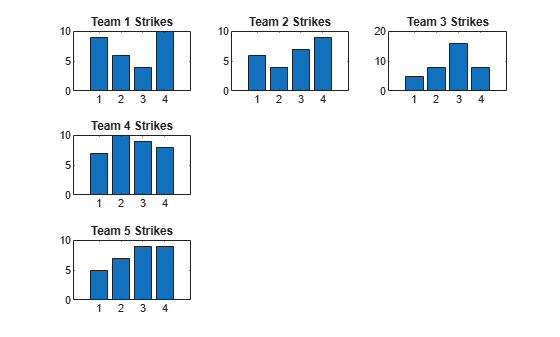Display a larger plot with a legend. Call the `nexttile` function to place the upper left corner of the axes in the fifth tile, and span the axes across two rows by two columns of tiles. Plot the scores for all the teams. Configure the x-axis to display four ticks, and add labels to each axis. Then add a shared title at the top of the layout.

```nexttile(5,[2 2]); plot([1 2 3 4],scores,'-.') labels = {'Team 1','Team 2','Team 3','Team 4','Team 5'}; legend(labels,'Location','northwest') % Configure ticks and axis labels xticks([1 2 3 4]) xlabel('Game') ylabel('Score') % Add layout title title(t,'April Bowling League Data')```Create a 1-by-2 tiled chart layout. In the first tile, display a geographic plot containing a line that connects two cities on a map. In the second tile, create a scatter plot in polar coordinates.

```tiledlayout(1,2) % Display geographic plot nexttile geoplot([47.62 61.20],[-122.33 -149.90],'g-*') % Display polar plot nexttile theta = pi/4:pi/4:2*pi; rho = [19 6 12 18 16 11 15 15]; polarscatter(theta,rho)```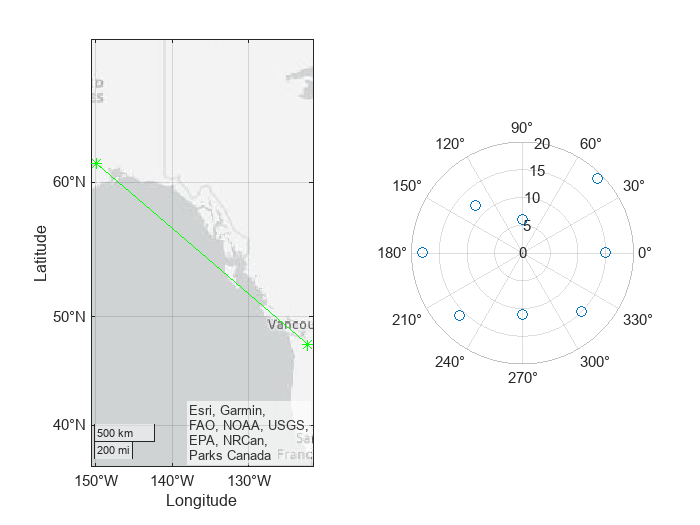One of the ways that the `nexttile` output argument is useful is when you want to adjust the content in a previous tile. For example, you might decide to reconfigure the colormap used in a previous plot.

Create a 2-by-2 tiled chart layout. Call the `peaks` function to get the coordinates for a predefined surface. Then create a different plot of the surface in each tile.

```tiledlayout(2,2); [X,Y,Z] = peaks(20); % Tile 1 nexttile surf(X,Y,Z) % Tile 2 nexttile contour(X,Y,Z) % Tile 3 nexttile imagesc(Z) % Tile 4 nexttile plot3(X,Y,Z)```To change the colormap in the third tile, get the axes in that tile. Call the `nexttile` function by specifying the tile number, and return the axes output argument. Then pass the axes to the `colormap` function.

```ax = nexttile(3); colormap(ax,cool)```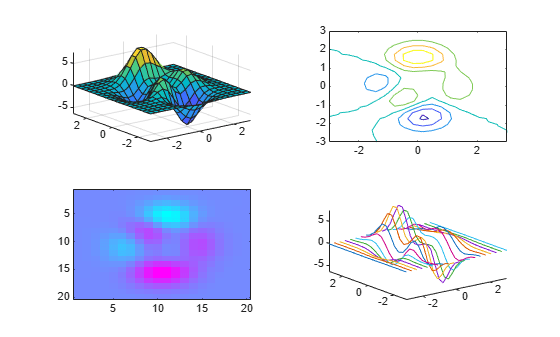Create a 2-by-3 tiled chart layout containing two plots in individual tiles, and one plot that spans across two rows and two columns.

```t = tiledlayout(2,3); [X,Y,Z] = peaks; % Tile 1 nexttile contour(X,Y,Z) % Span across two rows and columns nexttile([2 2]) contourf(X,Y,Z) % Last tile nexttile imagesc(Z)```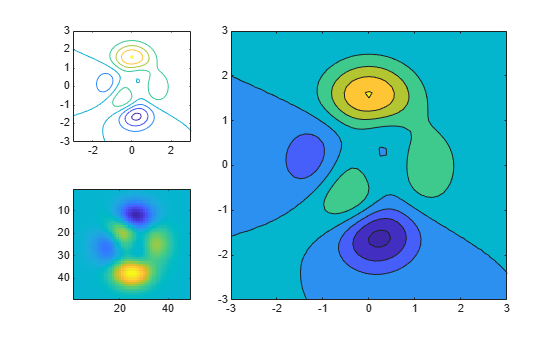To change the colormap for the spanned axes, identify the tile location as one containing the upper-left corner of the axes. In this case, the upper-left corner is in the second tile. Call the `nexttile` function with `2` as tile location, and specify an output argument to return the axes object at that location. Then pass the axes to the `colormap` function.

```ax = nexttile(2); colormap(ax,hot)```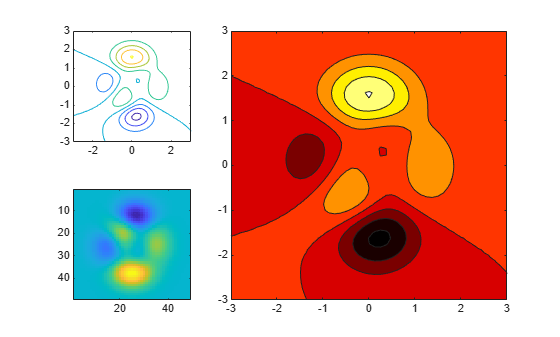Load the `patients` data set and create a table from a subset of the variables. Then create a `2`-by-`2` tiled chart layout. Display a scatter plot in the first tile, a heatmap in the second tile, and a stacked plot across the bottom two tiles.

```load patients tbl = table(Diastolic,Smoker,Systolic,Height,Weight,SelfAssessedHealthStatus); tiledlayout(2,2) % Scatter plot nexttile scatter(tbl.Height,tbl.Weight) % Heatmap nexttile heatmap(tbl,'Smoker','SelfAssessedHealthStatus','Title','Smoker''s Health'); % Stacked plot nexttile([1 2]) stackedplot(tbl,{'Systolic','Diastolic'});```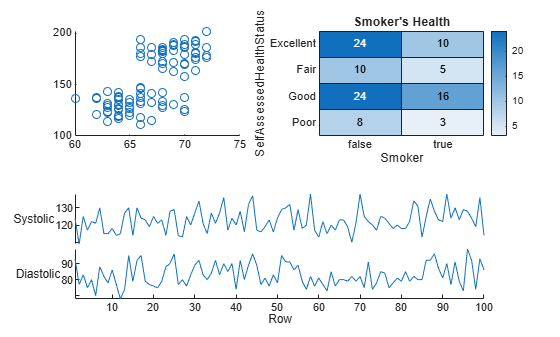Call `nexttile`, and specify the tile number as `1` to make the axes in that tile the current axes. Replace the contents of that tile with a scatter histogram.

```nexttile(1) scatterhistogram(tbl,'Height','Weight');```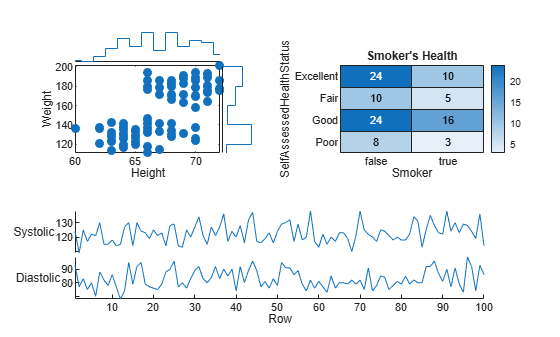When you want to share a colorbar or legend between two or more plots, you can place it in a separate tile.

Create filled contour plots of the `peaks` and `membrane` data sets in a tiled chart layout.

```Z1 = peaks; Z2 = membrane; tiledlayout(2,1); nexttile contourf(Z1) nexttile contourf(Z2)```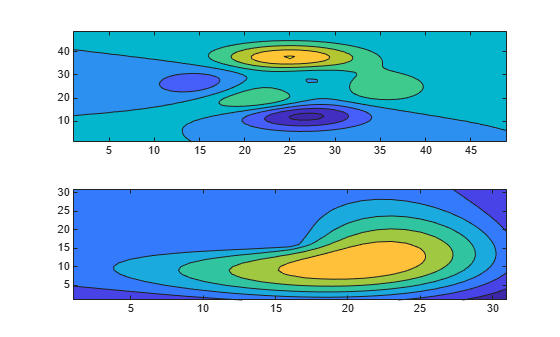Add a colorbar, and move it to the east tile.

```cb = colorbar; cb.Layout.Tile = 'east';```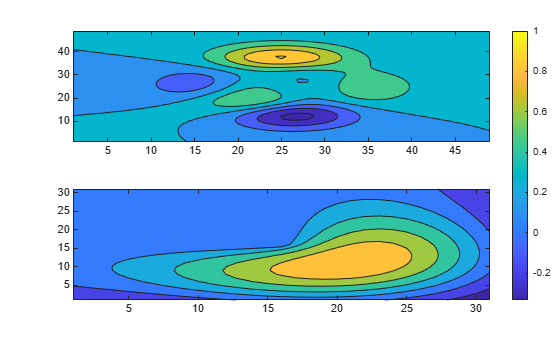Occasionally, you might need to create the axes manually before calling a plotting function. When you create the axes, specify the `parent` argument as the tiled chart layout. Then position the axes by setting the `Layout` property on the axes.

Create a tiled chart layout `t` and specify the `'flow'` tile arrangement. Display a plot in each of the first three tiles.

```t = tiledlayout('flow'); nexttile plot(rand(1,10)); nexttile plot(rand(1,10)); nexttile plot(rand(1,10));```Create a geographic axes object `gax` by calling the `geoaxes` function and specify `t` as the parent argument. By default, the axes goes into the first tile, so move it to the fourth tile by setting `gax.Layout.Tile` to `4`. Span the axes across a `2`-by-`3` region of tiles by setting `gax.Layout.TileSpan` to `[2 3]`.

```gax = geoaxes(t); gax.Layout.Tile = 4; gax.Layout.TileSpan = [2 3];```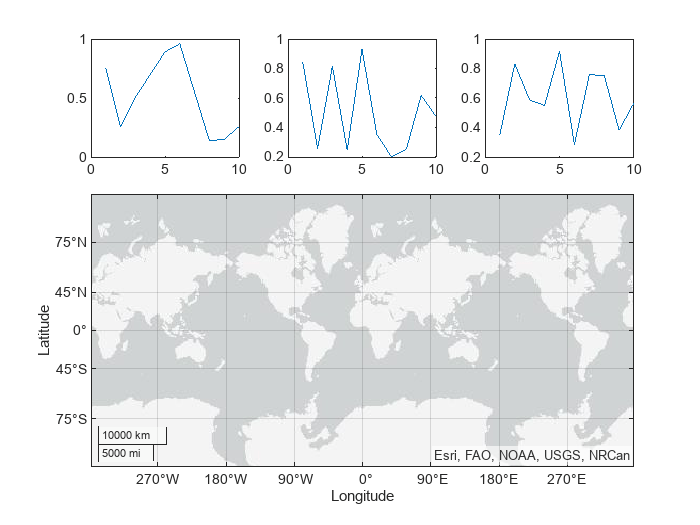Call the `geoplot` function. Then configure the map center and zoom level for the axes.

```geoplot(gax,[47.62 61.20],[-122.33 -149.90],'g-*') gax.MapCenter = [47.62 -122.33]; gax.ZoomLevel = 2;```## Input Arguments

collapse all

Number of rows, specified as a positive whole number.

Example: `tiledlayout(2,3)` creates a tiled chart layout that has two rows and three columns of tiles.

Number of columns, specified as a positive whole number.

Example: `tiledlayout(2,3)` creates a tiled chart layout that has two rows and three columns of tiles.

Parent container, specified as a `Figure`, `Panel`, `Tab`, `TiledChartLayout`, or `GridLayout` object.

### Name-Value Pair Arguments

Specify optional comma-separated pairs of `Name,Value` arguments. `Name` is the argument name and `Value` is the corresponding value. `Name` must appear inside quotes. You can specify several name and value pair arguments in any order as `Name1,Value1,...,NameN,ValueN`.

Example: `tiledlayout(2,2,'TileSpacing','compact')` creates a 2-by-2 layout that has minimal spacing between the tiles.

Note

The properties listed here are only a subset. For a complete list, see TiledChartLayout Properties.

Tile spacing, specified as `'loose'`, `'compact'`, `'tight'` or `'none'`. Use this property to control the spacing between the tiles.

This table shows how each value affects the appearance of a `2`-by-`2` layout.

ValueAppearance

`'loose'`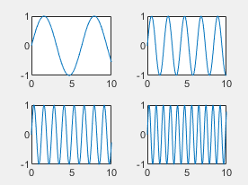`'compact'`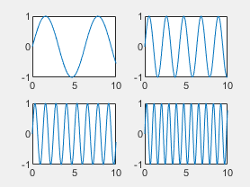`'tight'``'none'`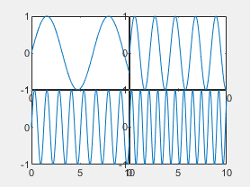Padding around the perimeter of the layout, specified as `'loose'`, `'compact'`, or `'tight'`. The layout provides space for all decorations, such as axis labels, regardless of the value of this property.

This table shows how each value affects the appearance of a `2`-by-`2` layout.

ValueAppearance

`'loose'``'compact'`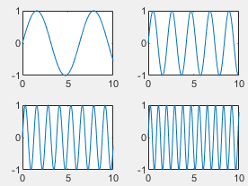`'tight'`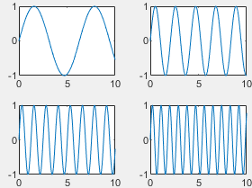## Compatibility Considerations

expand all

Behavior changed in R2021a

## See Also

### Properties

Introduced in R2019b

Download ebook